# Simple Harmonic Motion

Simple harmonic motion is typified by the motion of a mass on a spring when it is subject to the linear elastic restoring force given by Hooke's Law. The motion is sinusoidal in time and demonstrates a single resonant frequency.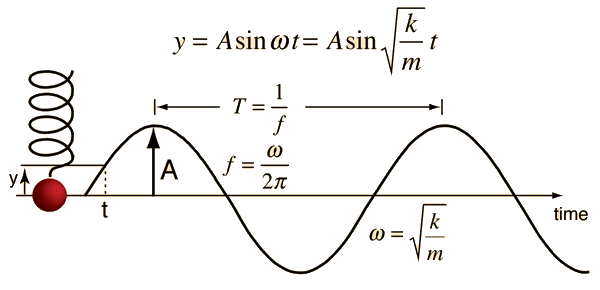Motion equations Motion calculation Frequency calculation Motion sequence visualization
 Damped oscillation Driven oscillation
Index

Periodic motion concepts

 HyperPhysics***** Mechanics R Nave
Go Back

# Simple Harmonic Motion Equations

The motion equation for simple harmonic motion contains a complete description of the motion, and other parameters of the motion can be calculated from it.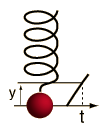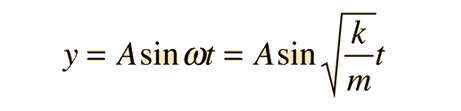The velocity and acceleration are given by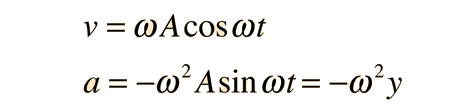The total energy for an undamped oscillator is the sum of its kinetic energy and potential energy, which is constant at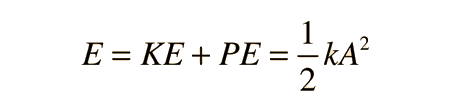Energy transformation in periodic motion
Index

Periodic motion concepts

 HyperPhysics***** Mechanics R Nave
Go Back

# Simple Harmonic Motion Calculation

The motion equations for simple harmonic motion provide for calculating any parameter of the motion if the others are known.If the period is T =s
then the frequency is f = Hz and the angular frequency = rad/s.

The motion is described by

Displacement = Amplitude x sin (angular frequency x time)
 y = A x sin ( ω x t ) m = m x sin ( rad/s x s )

Any of the parameters in the motion equation can be calculated by clicking on the active word in the motion relationship above. Default values will be entered for any missing data, but those values may be changed and the calculation repeated. The angular frequency calculation assumes that the motion is in its first period and therefore calculates the smallest value of angular frequency which will match the other parameters. The time calculation calculates the first time the motion reaches the specified displacement, i.e., the time during the first period.

Index

Periodic motion concepts

 HyperPhysics***** Mechanics R Nave
Go Back